OR Function Examples – Excel & Google Sheets

This tutorial demonstrates how to use the Excel OR Function in Excel to test if one or more criteria is true.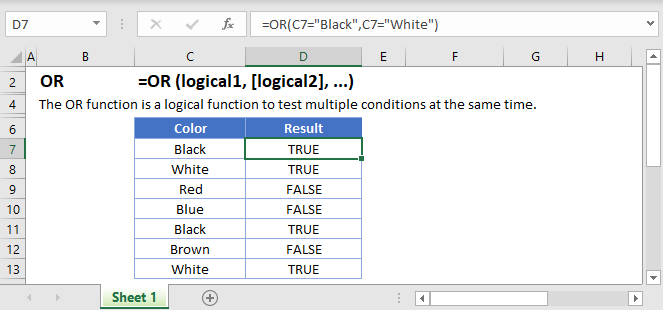OR Function Overview

The OR Function Checks whether any conditions are met. Returns TRUE or FALSE.

To use the OR Excel Worksheet Function, select a cell and type: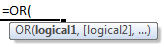(Notice how the formula inputs appear)

OR function Syntax and inputs:

logical1 – Logical expressions. Example: A1 > 4.

What is the OR Function?

OR is one of Excel’s logical functions. It evaluates several expressions, and returns TRUE if any one of the conditions are true. OR can evaluate up to 255 expressions.

How to Use the OR Function

Use the Excel OR Function like this: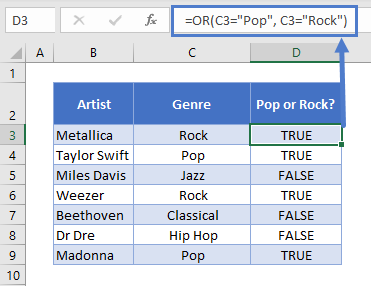If the text in column C is equal to “Pop” or “Rock”, OR will return TRUE. Anything else, and it returns FALSE.

Comparing Text

Note that text comparisons are not case-sensitive. So the following formula would produce the same results as above: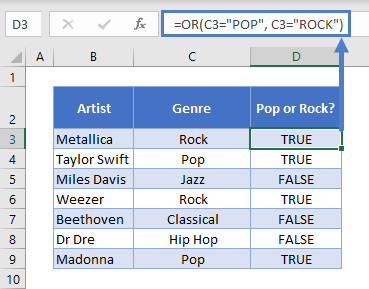Also, OR does not support wildcards. So this formula returns FALSE: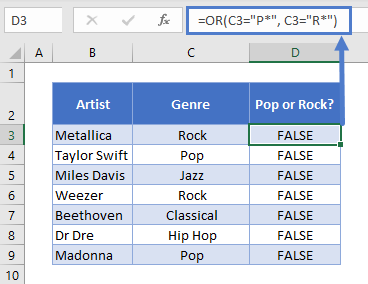This is because OR will literally compare the text string “P*” and “R*” with the value in C3.

Comparing Numbers

You have a range of comparison operators at your disposal when comparing numbers. These are: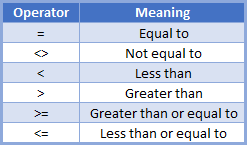For example, if you had a list of 1980s movies and wanted to find ones from 1983 and earlier, or 1987 and later, you could use this formula: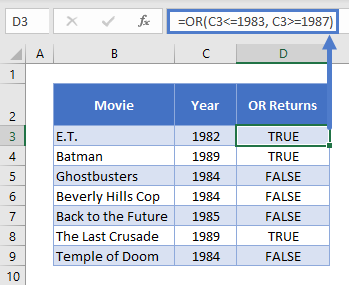If one of the expressions in OR is a number by itself without a comparison operator, such as =OR(1983), OR will return TRUE for that value, except if the number is zero, which evaluates to FALSE.

Using OR with Other Logical Operators

You can combine OR with any of Excel’s other logical operators, such as AND<<link>>, NOT<<link>>, and XOR<<link>>.

Here’s how you might combine it with AND. Imagine we have a table with data on some movies. We want to find movies released after 1985 that were directed by either Steven Spielberg or Tim Burton. We could use this formula:

•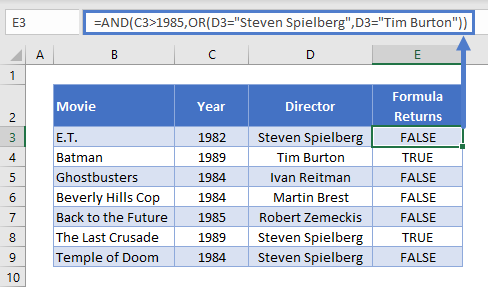When you combine logical operators in this way, Excel works from the inside-out. So it will evaluate the OR statement here first, since that’s nested within the AND.

Using OR with IF

OR is most commonly used as part of a logical test in an IF statement.

Use it like this: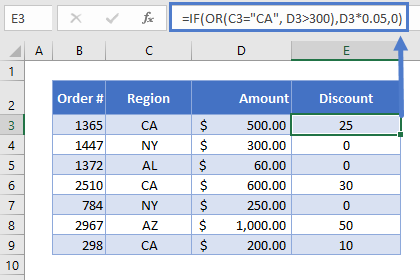Imagine we’re running some new promotions. To help us break into the California market, we’re offering a 5% discount in that state. We’re also offering 5% off any order over \$300, to encourage our customers to make bigger orders.

The IF statement evaluates our OR Function first. If returns TRUE, IF will return D3*0.05, which give us the 5% discount value. If not, it returns 0: the order didn’t meet our criteria, so we don’t apply the discount.

Read more on the main page on the Excel IF Function <<link>>.

The OR Function works exactly the same in Google Sheets as in Excel: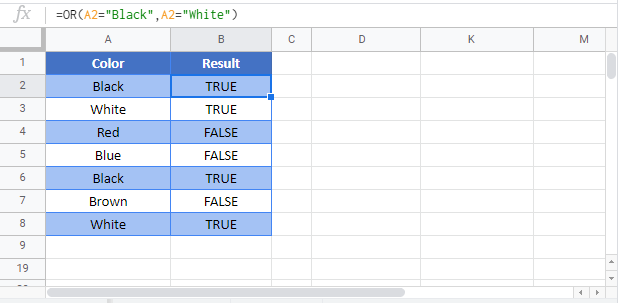Use the OR Function to test if one or more conditions are met. Each condition must be a logical expression (ex: a1 >5), a reference to a cell containing TRUE or FALSE, or an array containing all logical values. If one or more conditions are TRUE, the formula returns TRUE, otherwise it returns FALSE.

OR is a logical function and is generally used in conjunction with another logical function, IF:
The IF Formula does one thing if a condition is TRUE and does another if a condition is FALSE. Often when using the OR Function you will “nest” it within an IF Function.

You may also want to use these other logical functions:
AND Formulas test if all conditions are met.
XOR Formulas test if one and only one condition are met.

OR Examples in VBAYou can also use the OR function in VBA. Type:
Application.Worksheetfunction.Or(logical1,logical2)
For the function arguments, you can either enter them directly into the function, or define variables to use instead.